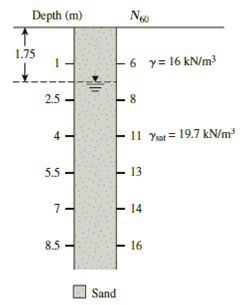Chapter 17, Problem 17.9PPrinciples of Geotechnical Enginee...

9th Edition
Braja M. Das + 1 other
ISBN: 9781305970939

Solutions

Chapter
SectionPrinciples of Geotechnical Enginee...

9th Edition
Braja M. Das + 1 other
ISBN: 9781305970939
Textbook Problem

Refer to Figure 17.16. Estimate the variation of cone penetration resistance, qc, with depth, using Eq. (17.39) and values of c and a given by Kulhawy and Mayne (Table 17.7). Assume D50 = 0.46 mm.17.7 Refer to the boring log shown in Figure 17.16. Estimate the average drained friction angle, ϕ ′ , using the Kulhawy and Mayne correlation [Eq. (17.24)]. Assume pa ≈ 100 kN/m2.Figure 17.16

To determine

Find the variation of cone penetration resistance with various depth.

Explanation

Given information:

The atmospheric pressure is (pa) 100kN/m2.

Assume the grain size (D50) 0.46mm.

Show the depth and number of blows shown in table:

 Depth,(m) N60 1 6 2.5 8 4 11 5.5 13 7 14 8.5 16

Calculation:

Show the expression of correlation between qcandN60 using the Equation:

(qcpa)N60=cD50a (1)

Here, pa is atmospheric pressure, N60 is standard penetration resistance, c and a as developed from various studies.

For c and a value given by Kulhawy and Mayne(1990).

Refer Table 17.7 in section 17.11 “Cone penetration test” in the textbook.

 c a 5.44 0.26

Find the variation of cone penetration resistance with various depth 1m

Substitute 100kN/m2 for pa, 0.46mm for D50, 5.44 for c, 0.26 for a, and 6 for N60 in Equation (1).

(qc100)6=5.44(0.46)0.26(qc100)6=4.4454(qc100)=26.67qc=2,667kN/m2

Thus, the variation of cone penetration resistance at the depth 1m is 2,667kN/m2_.

Find the variation of cone penetration resistance with various depth 2.5m.

Substitute 100kN/m2 for pa, 0.46mm for D50, 5.44 for c, 0.26 for a, and 8 for N60 in Equation (1).

(qc100)6=5.44(0.46)0.26(qc100)8=4.4454(qc100)=35.56qc=3,556kN/m2

Thus, the variation of cone penetration resistance at the depth 2.5m is 3,556kN/m2_.

Find the variation of cone penetration resistance with various depth 4m.

Substitute 100kN/m2 for pa, 0.46mm for D50, 5.44 for c, 0.26 for a, and 11 for N60 in Equation (1).

(qc100)6=5.44(0.46)0.26(qc100)11=4

Still sussing out bartleby?

Check out a sample textbook solution.

See a sample solution

The Solution to Your Study Problems

Bartleby provides explanations to thousands of textbook problems written by our experts, many with advanced degrees!

Get Started

What does WCS stand for?

Precision Machining Technology (MindTap Course List)

Define each of the following terms: a. data b. field c. record d. file

Database Systems: Design, Implementation, & Management

What is projectitis? How is it cured or its impact minimized?

Principles of Information Security (MindTap Course List)

Discuss the importance of data models.

Database Systems: Design, Implementation, & Management

Describe the strategy of termination.

Management Of Information Security

Define an object, and provide three examples.

Systems Analysis and Design (Shelly Cashman Series) (MindTap Course List)

What is a catalyst?

Automotive Technology